# Spirals of Creation

The story of creation begins with Source.  This is what makes sense of everything.  It is the great story of how Source in its infinite freedom, aliveness and creativity creates structure within itself and experiences this structure as a means of exploring its own nature.  We are an integral part of this process – it is our story too.  We are creation in action.  Spirals are a significant structure in the whole dynamic of creation.  They provide a fascinating cross section across many levels of creation and help illustrate the beauty, scale and profundity of the vast cosmic structure we are part of.

We will explore a little math (geometry and number) and science along the way.  This helps us appreciate the sheer depth, coherence, integrity and power of this vast and wonderful body of knowledge.  It is an exciting journey that explores the larger reality we live in and ultimately helps us explore our own nature as it connects all the way back to Source.

The spirals information in this document is based on the original definition of the Krystal Spiral translated by E’Asha Ashayana from Eieyani sources and presented in Reference 8 and in subsequent workshop materials.  Krystic Merkaba information and symbol codes also derive from these sources.  This is gratefully acknowledged.  Please refer to the References listed at the end for more detailed background information.  The active guidance, support and assistance of ASaN Deane and Wilbur contributed immensely to the development of this information and is also gratefully acknowledged.  Likewise, the Kathara Team provided immense support and facilitation.

## Krystal Spiral

The Krystal spiral is the primary spiral that emerges from Source and carries the original creation encryption of the Kryst Code, hence the name Krystal Spiral.  The Kryst Code embodies Source’s original intention for creation.  This is expressed or carried into manifestation through the primal template of the Kathara Grid.  The Kathara Grid in turn generates the Krystal Spiral, which facilitates expansion into creation and ultimate re-integration back to Source.

The Kathara Grid is a key structure that carries the encryption or template for manifestation in 12 dimensions of creation.  It comprises 12 centers, one relating to each dimension, interconnected by a grid of lines in a very precise geometric pattern as illustrated in the diagram.  A key line is the central vertical line or column running through the grid between centers 1 and 12.  Krystic structures have a central point that provides a doorway for direct connection to Source.  The Kathara Grid has such a Central Seed Atom (CSA), also known as the AzurA point, at the mid point on the central vertical column.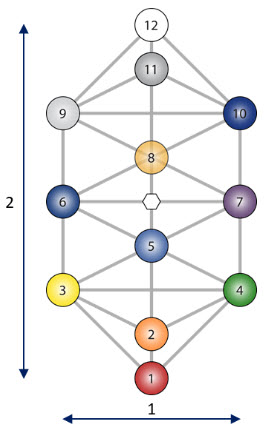The proportions of the Kathara Grid are important as these condition the patterns that are subsequently built on the grid.  The key dimensions are the length of the central vertical column between centers 1 and 12 and the width between centers 6 and 7.  These are in the exact proportion of 2 to 1.  The arrangement of the centers, their distances and angular alignments are also important as these condition the interaction between the centers.

The Krystal Spiral is generated by rotating and expanding the Kathara Grid.  The Kathara Grid is rotated about its AzurA center so that the center 9 to AzurA line of the current grid overlays the center 12 to AzurA line of the previous grid.

This progression is illustrated in the diagrams over.  We start with the Kathara Grid in Stage 0.  This is in alignment with the Ecka axis in our universe.  The Kathara Grid rotates from the yellow into the vertical blue position in Stage 1.  The Kathara Grid also expands so that the new 9 center overlays the prior 12 position.

In Stage 2 the Kathara Grid continues its rotation into the clear position.  Here again the new 9 center overlays the prior 12 position.  Also the new 6 center overlays the 12 position of two stages back i.e. Stage 0.  The Kathara Grid continues rotating and expanding in this fashion.

The 12 center generates the Krystal Spiral as the Kathara Grids rotate.  If we join the center 12 positions from each stage we get the Krystal Spiral as illustrated in blue in the diagram over.  This shows expansion out to Stage 9.  Only the outlines of the earlier/inner Kathara Grids are shown for clarity.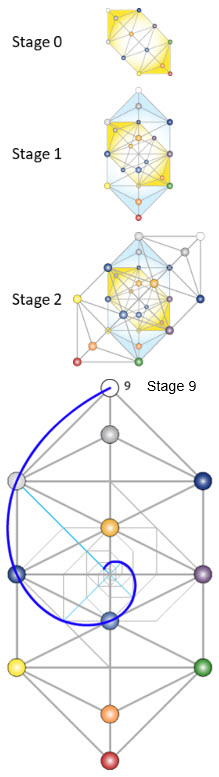Here we see that all the Kathara Grids hold connection at the same AzurA point.  This is turn holds connection to Source for every stage.  This geometric center is the pivot point for the grids.  It is also the center for the spiral.  The 12 centers that generate the spiral connect to the AzurA center along the central vertical column of the grids.  These connection lines form arms or radii for the spiral and are illustrated in light blue in the diagram.  The spiral is connected thereby to the Source center at every stage.

Geometrically every cycle of expansion entails a rotation through 45° and an increase in size by √2 (square root of 2 = 1.414…).  Going through two stages, as from Stage 0 to 2 above, involves rotation through 90° and a doubling of size.  Effectively the Kathara Grid length equates to the width two stages ahead.  Here we see how the Kathara Grid proportions determine the scaling of the spiral.  The expansion by 2 comes directly from the Kathara Grid.

This process illustrates the formation of the spiral in a clockwise direction to generate what is referred to as the EtorA Krystal Spiral.  There is a complementary spiral that forms from the same starting position but rotates in the counter-clockwise direction to generate an AdorA Krystal Spiral.  This is a mirror image of the spiral above.  The two spirals interact to form other structures as represented in the Rosetta Code for example.

The proportions associated with the spiral for every 90° or quadrant of expansion are illustrated in the diagram over, i.e. there is a doubling every quadrant.  This gives us the number sequence – 1, 2, 4, 8, 16, etc.  There is repeated multiplication by 2.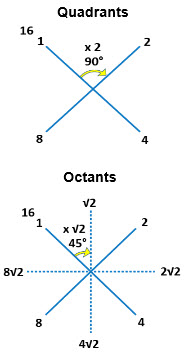The √2 expansion between 45° stages can be regarded as intermediate or half steps between the quadrants.  This gives us octant stages as illustrated in the lower diagram with the corresponding numbers.  The spiral, of course, is continuous and passes through every number.  The numbers at specific stages of expansion are, however, of particular interest.

The number 2 is key to the Krystal Spiral.  √2 is also significant and it’s worth looking at where this comes from.  Multiplying a number by itself, e.g. 2 x 2 = 4, is referred to as squaring, as this gives us the area of a square with sides that length.  If we start with the area and wish to find the length we reverse the process to find the square root (√) of the number, e.g. what number multiplied by itself would give 4.  In this case the answer is relatively simple, √4 = 2.  If we start with 2 however, as illustrated in the diagram over, the result isn’t so tidy but is still valuable.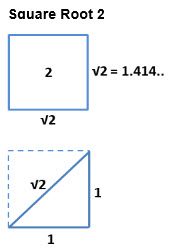The number that we would need to multiply by itself to get 2 is √2 = 1.414…  The decimal value continues indefinitely, but the finer detail is of less importance.

Geometrically we also get this number as the diagonal of a square.  If we take a square of side 1 for example it will have a diagonal of length √2, as illustrated in the lower diagram.  This provides a convenient way of measuring this number.

Mathematically the square root (√) can be regarded as a half step in multiplication.  For example to split multiplying by 2 into two stages we would multiply by √2 = 1.414…and again by this same number to get the 2 result, i.e. √2 x √2 = 2.

We see therefore that the spiral entails both rotation and expansion by multiplication.  Repeated multiplication is referred to as exponentiation.  The Krystal Spiral forms a smooth exponential spiral that looks the same at every scale from the microcosmic all the way to the macrocosmic.

## Krystal Spiral in Veca Universe

We can see a good example of the Krystal Spiral in operation in the structure of a Veca universe

The Kathara Grid structures in a Veca Universe are illustrated in the diagram over.  There are definite size relationships and orientations between the different Kathara Grids.

The inner Ecka core (red) is 2x√2 times smaller than the Density and Primal Grids (grey) in the 4 quadrants.  The outer Eckasha Grid (blue) is 2x√2 times larger than the Density and Primal Grids (grey).  The Eckasha is therefore 8 times bigger then the Ecka core.  With 270° of clockwise rotation this corresponds to 3 stages of quadrant spiral expansion.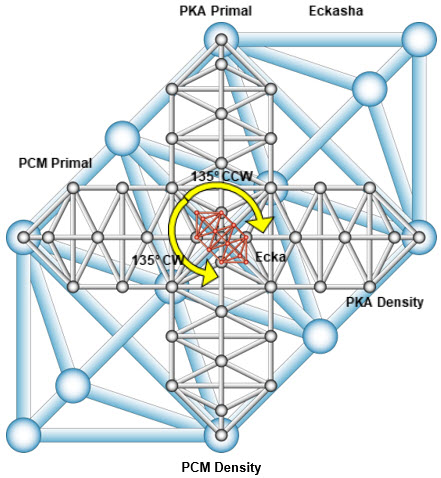Likewise the Density and Primal Grids have 135° of rotation both clockwise and counter-clockwise from the Ecka core.  It can be noted that the Ecka core comprises two Kathara Grids overlaid on each other.  With the 2x√2 expansion in size this corresponds to 3 stages of octant expansion.  The Krystal Spiral therefore conditions the structure and expansion of a Veca universe.

## Golden Mean

The Golden Mean is a specific number obtained as follows.  Divide a line so that the ratio or proportion between the whole and the larger portion is the same as that between the larger and the smaller portions.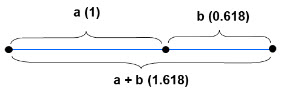So in the diagram above ‘a’ is the larger and ‘b’ the smaller portions of a line.  ‘a + b’ is the whole.  Then

(a + b) / a  =   a / b  =  Golden Mean  =  Phi (Φ).

The ratio or proportion between the numbers is referred to as the Golden Mean.  This number is commonly denoted by the Greek letter Phi (Φ).  With a little math we can determine this number Φ  =  1.618…  The decimals continue indefinitely but the finer detail is of lesser importance.

This means that if we take the larger portion of the line as  a = 1 in the diagram above the whole line will be 1.618… and the smaller portion b = 0.618…  Then

1 / 0.618…  =  1.618…

This also means that if we take a sequence of numbers (or objects) stepping up in size from one stage to the next by this proportion of 1.618… then each number will be the sum of the previous two.  This property is what defines the Golden Mean.

Also if we take a sequence of numbers where each term is the sum of the previous two the ratio or proportion between successive numbers will move towards the Golden Mean value of Φ.  This can be any sequence, e.g. 12, 15, 27, 42, 69, etc. provided each term is the sum of the previous two.  It may take a while for the pattern to settle down, but the proportion will progressively converge towards Φ.

With math we can also express the number in the following form:

Φ   =   1.618…   =   (√5  +  1) / 2

This illustrates that the number can be obtained geometrically as follows.  Take a rectangle with sides 2 x 1, i.e. in the proportion 2 : 1, as illustrated in the diagram.  The diagonal of this rectangle is √5.  We can get the number Φ by adding the diagonal and the short side of this rectangle and then dividing this by the long side.  Alternatively if we start with the long side of the rectangle as 1 and the short side as ½ then adding the diagonal and the short side gives us Φ directly.  This process is analogous to using a square to obtain the √2 for the Krystal Spiral above, although a bit more complex.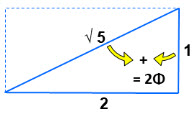We also see that Φ is heavily associated with the number 5.  The root sound tones are similar – five, phi and Fibonacci, which we will come across shortly.  The Φ ratio is present in all regular 5 sided figures, the pentagon, pentagram, etc.  For example the width (distance between opposite corners) across a pentagon is in Φ ratio to the sides, as illustrated in the diagram.  The number Φ is needed to construct a regular pentagon.  The ratios of successive lengths in a pentagram (5-pointed star) are Φ and so on.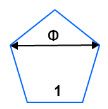More generally, the term Golden Mean originally referred to a path that avoided extremes in either direction.  Apparently the term was only applied to the Φ ratio in the last few hundred years.

## Golden Rectangle

The next stage in the progression towards a Golden Mean (GM) spiral is to construct a Golden Rectangle on the golden mean line above.  We can accomplish this by taking a square of side 1 as illustrated in the diagram below.

Halve one side at ‘a’.  Then take a diagonal from ‘a’ to an opposite corner ‘D’ and rotate this down onto the halved side.  The resulting line ‘a – E’ will extend outside the original square so that the line ‘A – B – E’ is in Golden Mean proportion with the sides of the square..

Complete the rectangle ‘A – E – F – D’ and we have a Golden Rectangle.  The length ‘A –E’ is 1.618… and the width or height ‘A – D’ is 1.  The rectangle therefore has a length to width ratio of Φ  = 1.618… and is in Golden Mean proportion.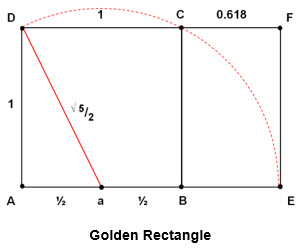The Golden Rectangle comprises two elements.  We have the original square ‘A – B – C – D’.  We now also have an additional smaller rectangle ‘B – E – F – C’.  This is also a golden rectangle in Φ proportion, i.e. the ratio of the sides ‘BC : BE’ is 1.618…  The larger golden rectangle is scaled exactly Φ times bigger than the smaller embedded one.  It is also rotated 90° relative to it.

## Golden Mean Spiral

Now if we take the golden rectangle above and form another square on the long side ‘A – E’ we get a new golden rectangle.  This will have a width 1.618… and a length of 1.618… + 1  =  2.618…  These numbers and corresponding rectangle sides are in Golden Mean proportion.  Effectively we have a new golden rectangle, which is scaled Φ = 1.618… times bigger than that above and rotated 90° relative to it.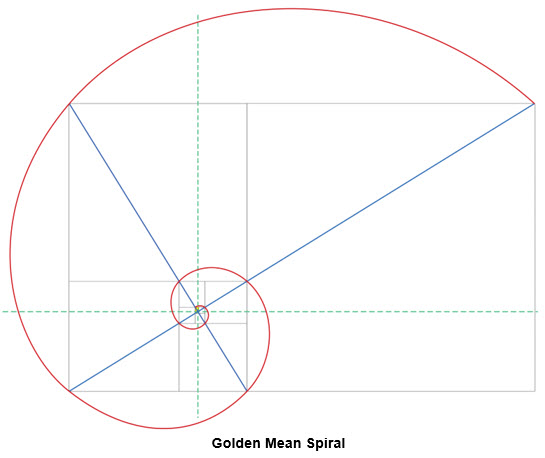We can now form another bigger square on the long side of the latest Golden Rectangle to get another Golden Rectangle that is Φ times bigger again and rotated through 90°.  We can continue this process indefinitely.  At each stage the squares and rectangles increase in proportion by Φ as they rotate through 90°.  It is now possible to join the outer corners of the squares to form a spiral.  This is known as the Golden Mean (GM) spiral and is illustrated in the diagram above.  It is a smooth exponential spiral, expanding by Φ for every rotation through 90°.  The spiral can rotate clockwise or counter-clockwise depending on the direction we choose at the start.

The traditional means of viewing the Golden Mean spiral is as a sequence of squares expanding around a small golden rectangle at the core.  This is evident in the diagram above.

However, it is also being created by a similar sequence of expanding Golden Rectangles.  This gives a much more powerful insight into what is happening.  The Golden Rectangles overlap and rotate around a common pivot point.  We can see this if we examine the Golden Rectangles in the following sequence of diagrams.  The rectangles are colored yellow as they increase progressively, stage by stage.

Now if we look closely at the point where the blue lines cross we can see that this point is proportionately in the same location for each Golden Rectangle.  As the rectangles expand they rotate 90° around this fixed pivot point.  Allowing for the rotation this pivot point is proportionately in the same location for each rectangle, i.e. about 28% in from the corner opposite the spiral point.

Furthermore, if we were to continue the spiral inwards it would progressively circle around this point and converge towards it, but only reaching it after an infinite number of cycles.  So this is the center point for the Golden Mean spiral.  It is also the fixed pivot point for the rectangles, as they rotate to generate the spiral.  It is a key power point in the Golden Rectangle.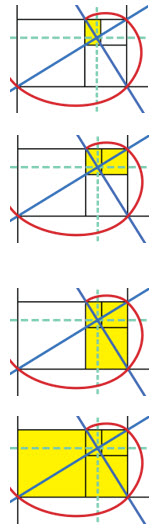We can find this pivot point within the Golden Rectangle as follows.  With reference to the rectangle diagram below draw a diagonal ‘A – F’ for the large rectangle.  Draw another one ‘C – E’ for the small inner Golden Rectangle as illustrated in the diagram.  These diagonals intersect at the pivot point ‘O’.  This is the center for the Golden Mean spiral.

With ‘O’ as a center, the diagonal elements ‘O – C’, ‘O – F’, and so on now form the arms or radii for the spiral.  These radii are important, as they measure the distance from the center at each point of expansion.  They lie at 90° to each other and increase in size by Φ = 1.618…  The spiral passes through points ‘C, F, E, A’, etc. as it expands outwards and onto the next level.

The position of this pivot / center point ‘O’ can be calculated from the geometry.  It is located 0.7236… of the way in along the sides of the rectangle.  In other words ‘A – x’ is about 72% of the length of the long side ‘A – E’, while ‘A – y’ is similarly about 72% of the length of the short side ‘A – D’.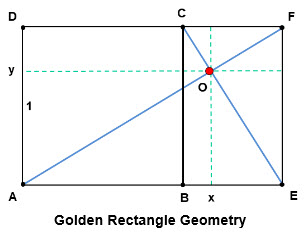We now see a pattern of behaviour somewhat similar to the formation of the Krystal spiral.  We have a sequence of Golden Rectangles rotating about a common pivot point and increasing in size as they rotate.  The pivot point is off center geometrically within the rectangles.  This differs from the symmetrical center in the Kathara grids for the Krystal spiral.  Also, the expansion for each rotation through 90° is by Φ = 1.618… for the Golden Mean spiral, in comparison with by 2 for the Krystal Spiral.

## Fibonacci Spiral

The Golden Mean Φ is an expansion ratio that allows each number in a sequence to be the sum of the previous two.  We can start with the simplest numbers 0 & 1 to generate such a sequence.  We add each pair of numbers to get the following number.  This gives us the following sequence of numbers:

0, 1, 1, 2, 3, 5, 8, 13, 21, 34, 55, 89, 144,

If we examine the numbers associated with the Golden Mean spiral we also find a similar sequence embedded within them.  These are referred to as the Fibonacci numbers.

These numbers can also be used to generate a spiral as follows.  Start with a square of side 1.  Place another square of side 1 beside it.  Build a square on the long side of this, i.e. with side 2. Place another square on the long side of this combination, i.e. with side 3.  Continue building squares in the same direction around the outside on the long side of the structure.  These will increase in size from 3 through 5, 8, 13, 21, etc. following the Fibonacci sequence.  As the squares expand outwards around the structure, the outer corners trace a spiral pattern as shown.  This is known as the Fibonacci spiral.

If diagonals are drawn across the structure at each stage we get a central pivot point with radii extending out to the spiral points at each stage of expansion.  These radii are at 90° to each other and, after the initial stages, increase by Φ = 1.618… for each step.  This pattern is similar to the Golden Mean spiral.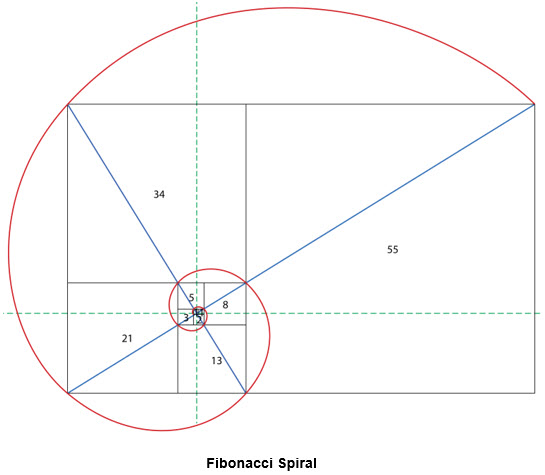It should be noted that in the early stages the Fibonacci curve isn’t a proper spiral.  Rotation implies rotation around a center and also curvature.  In the early stages, the center is moving around and the curvature is oscillating.  It settles on a stable center and smoothly changing curvature, as it aligns progressively with the shape of the Golden Mean spiral.  These are the center and curvature for the corresponding Golden Mean spiral.  Also an exponential spiral can in principle contract inwards past 1 and spiral inward towards its center.  The Fibonacci Spiral cannot do this.  It’s stuck at 1.

In particular the Fibonacci curve formed by drawing quadrant arcs within the squares isn’t a proper spiral – just a collection of arcs. That’s a convenience for ease of drawing.

## Spiral Numbers

We can now look at the number sequences for these spirals to discern further structure.  Starting from the base number 1 we take stages of 90° expansion.  1 is the starting point for multiplication sequences.  The Krystal Spiral in the second column is obtained by multiplying successively by 2.

The Golden Mean Spiral is built similarly by increasing each stage by Φ = 1.618…  This produces the sequence of numbers in the third column.

The Fibonacci Spiral sequence is shown in the fourth column.  Note that when we look at the Fibonacci sequence in terms of adding numbers we can consider it starting at 0.  However when we look at it geometrically in spiral terms it needs to start from 1.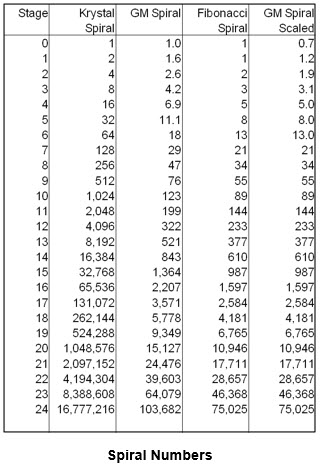We can see that the Krystal Spiral numbers increase much more rapidly than for the other spirals.  This indicates a stronger spiral.  We also see that the Golden Mean (GM) and the Fibonacci Spirals are not quite the same thing.  The GM Spiral numbers at each stage don’t match Fibonacci numbers.  The GM Spiral numbers do however satisfy the condition that the number at every stage is the sum of the previous two numbers.  This arises from the way the number Φ is defined.

The Fibonacci sequence is however related to the GM Spiral.  If we multiply the GM Spiral by a certain factor (= 0.7236…) we scale it down, so that it lines up more or less with the Fibonacci sequence.  This is shown in the 5th column in the table above.  Here we can see that the Fibonacci Spiral aligns closely with a scaled down version of the GM Spiral after the first few terms.  The Fibonacci Spiral effectively becomes a scaled version of the GM Spiral.

Where does this scaling factor of 0.7236… come from?  We have in fact seen this number already.  If we go back to the Golden Rectangle geometry above we see that the center/pivot point ‘O’ for the GM Spiral divides the rectangle in this same proportion.  The correspondence isn’t approximate.  It is absolutely exact.

## Fibonacci Rectangle

If we section the original golden rectangle at its pivot point ‘O’, as shown in the diagram over, we get a new Golden Rectangle scaled down by this 0.7236… factor.  This is shown in yellow.

This scaled rectangle now corresponds with the numbers and expansion sequence for the Fibonacci Spiral.  The numbers are 0.7236, 1.17, 1.9, 3.1, 5, 8, 13, …  These are the same as the numbers in the 5th column of the table above.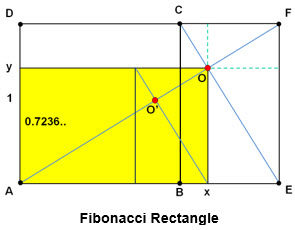So this scaled-down rectangle generates the Fibonacci Spiral just as the Golden Mean one generates the Golden Mean Spiral.  So this yellow rectangle effectively is the base Fibonacci rectangle.

We can find the inner square and smaller embedded Golden Rectangle within the Fibonacci Rectangle.  This allows the diagonals to be drawn to get the Fibonacci Spiral center and rectangle pivot point, i.e. at ‘O’.  Rotating and expanding this Fibonacci Rectangle can now generate the mature Fibonacci Spiral, just like the Golden Mean Spiral was generated.

We can see that the Fibonacci Spiral pulls its center in much closer to the true geometric center of the original Golden Rectangle.  The Golden Mean spiral center is quite a bit off center.

We now have a geometric connection between the Fibonacci and the Golden Mean Spirals.

## Fibonacci Spiral in relation to Kathara Grid

We can relate the Golden Mean and Fibonacci Spiral geometries back to the original Kathara Grid (KG), from which all emerged.  The Kathara Grid has a bounding rectangle represented by the width and height of the Kathara Grid.  This rectangle has a size ration of 2:1.  It is associated with the Krystal Spiral.  The Krystal Spiral has a center at the Central Seed Atom (CSA) at the center of the Kathara Grid, marked by the white hexagon in the diagram below.  The Krystal Spiral rotates around and expands from this center.

A golden rectangle can be generated within the KG rectangle, simply by taking one of the KG diagonal lines and rotating it in the vertical direction.  For example take the 3 – 7 diagonal in the KG diagram over and rotate it about the 3 center so that it lies vertically along the 3 – 6 – 9 line, extending past center 9.  This vertical line from the bottom of the KG to the end of the rotated diagonal is Φ = 1.618 times the width of the KG.  Completing a rectangle on this creates a Golden Rectangle within the KG.  This is illustrated by the green rectangle in the diagram below.  We could have used other diagonals to get a golden rectangle from the top down instead of the bottom up.

We can see that the Golden Rectangle excludes two dimensions, reducing the KG to 10 centers.  The Metatronic Tree of Life is similarly reduced from 12 to 10 centers.  This is discussed in greater detail below.  Interestingly, conventional physics theorizes that there are 10 dimensions in our local universe.

We can develop the geometry of the Golden Rectangle.  The lower half of the KG below the 6 – 7 line corresponds to the square within the Golden Rectangle.  The upper portion above the 6 – 7 line is itself a smaller Golden Rectangle within the main rectangle.  We can draw a diagonal for the main Golden Rectangle, e.g. from the corner above center 9 to the opposite corner, level with center 1.  We can also draw a diagonal for the smaller rectangle, e.g. from center 6 to the corner above center 10.  Their point of intersection, marked ‘GM’ in the diagram above, forms the center for a Golden Mean Spiral and the pivot point for a rotating sequence of Golden Rectangles as discussed previously.

We can now use the ‘GM’ pivot point to draw in the smaller Fibonacci Rectangle.  This is shown in yellow in the diagram below.  Again we can develop the geometry of the Fibonacci Rectangle to draw in its diagonals and their intersection point.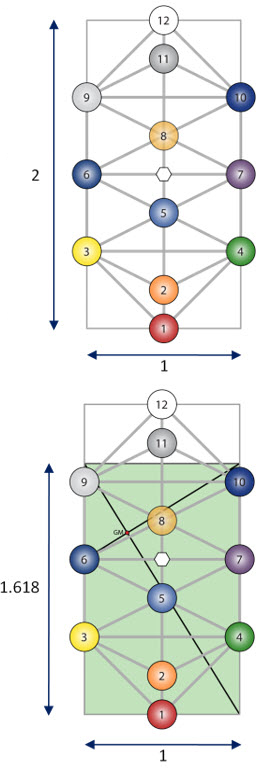This gives us the center for a Fibonacci Spiral, as marked by ‘Fib’ in the diagram.  A Fibonacci Spiral would rotate around and expand from such a pivot point.

Note that this Fibonacci center pulls in close to the central vertical column of the Kathara Grid.  In this position the Fibonacci Spiral center is impacting center 5 in the Kathara grid.

There are various ways we could have drawn these GM and Fibonacci Rectangles and their associated diagonals to get different placements and locations for the pivot points.  We get a particularly interesting set of alignments if we draw the Golden Mean Rectangle from the top of the KG downwards.  This is illustrated in the diagram below.  Here we have moved the set of Golden Mean and Fibonacci Rectangles into the upper portion of the KG.

In this case the Fibonacci Spiral center, marked ‘Fib’ and highlighted by the arrow in the diagram below, lies in extremely close alignment with Kathara center 8.  This is of huge interest as the 8 center is missing from the Metatronic Tree of Life.  The missing centers are 12 and 8.  These are key grid points.  Within the 15 dimensions of a local universe center 8 is the half way point and corresponds to the Galactic Core.  Center 12 is the connection point to the next level up in the cosmic structure.  In this instance the Fibonacci Spiral is rotating around the Galactic Core.

Also the Fibonacci Rectangle as shown embraces Kathara centers 4, 7 & 10 in addition to centers 5 & 8 afflicting them.  Center 4 is additionally afflicted by proximity to the major axis of both the GM and Fibonacci Rectangles.  Center 10 suffers similarly from proximity to the Fibonacci minor axis.  It’s possible to see how the 3 – 6 – 9 line would form a clearer passageway around this troubled region.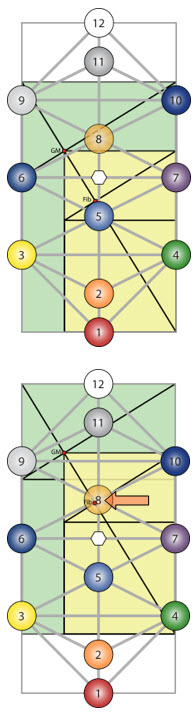If we draw in the Reuche Points on the original Kathara Grid we get some additional correspondences of interest.  These are illustrated in the diagram over.  Here we see that in addition to aligning with Kathara Center 8 the Fibonacci Spiral center ‘Fib’ also aligns with Reuche Point 12.  This is interesting in light of the missing 8 and 12 centers from the Metatronic Tree of Life.  Also the Golden Mean Spiral center ‘GM’ aligns with Reuche Point 10.

The three rectangles (Krystal, Golden Mean and Fibonacci) illustrate the relative size and power of the associated spirals.  They enshrine the spiral expansion ratios, i.e. Krystal Spiral – 2 : 1, Golden Mean spiral – 1.618.. : 1 and Fibonacci spiral – 1.618.. : 1 but starting from a scaled-down base.

There are other possible placements for the Golden Mean and Fibonacci Rectangles and their associated spiral centers within the original KG.  The second diagram below illustrates how the Fibonacci Spiral center ‘Fib’ also has an extremely close alignment with Kathara center 5.  Here the previous GM and Fibonacci rectangles are flipped top to bottom, so that they are coming from the bottom up, instead of the top down.  This illustrates how easily both Kathara centers 8 & 5 can be entrained by Fibonacci influences.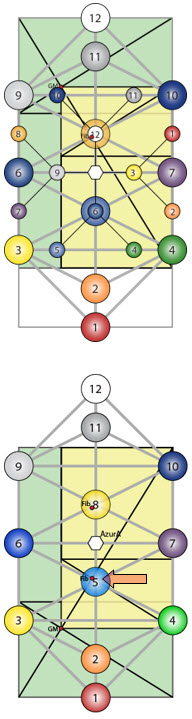## Krystal Spiral to Fibonacci Spiral

We can now trace a relationship from the Krystal Spiral to the Fibonacci Spiral in three stages as follows:

• Take the bounding rectangle for a Kathara Grid. This is in the ratio 2:1, i.e. length to width is 2:1.  Reduce the length to Phi = 1.618…  This produces a Golden Rectangle (green).
• Rotate and expand the Golden Rectangle so that the short edge of the current stage aligns with the long edge of the previous stage. This is similar to rotating the Kathara Grid to form the Krystal Spiral, except that for every 90° of rotation the Golden Rectangle expands by 1.618… vs. by 2 for the Krystal Spiral.  Also the Golden Rectangles are rotating around an off-center pivot point (GM).  This forms a Golden Mean Spiral centered around the off-center pivot point.
• Reduce the Golden Rectangle (green) to its embedded Fibonacci Rectangle (yellow). Rotate and expand the Fibonacci Rectangle, so that the short edge of the current stage aligns with the long edge of the previous stage.  This is similar to rotating the Kathara Grid to form the Krystal Spiral, except that for every 90° of rotation the Fibonacci Rectangle expands by 1.618… vs. by 2 for the Krystal Spiral.  The Fibonacci Rectangles are rotating around a somewhat more central pivot point (Fib), as shown.  This forms a Fibonacci Spiral rotating around the ‘Fib’ pivot point.

These stages are summarized in the following diagram: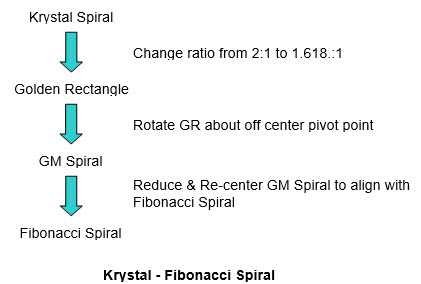This may not be how the Fibonacci Spiral actually was created.  However this sequence does give us a chain of connection back to the Krystal Spiral.  This is of considerable significance.

This provides significant information on center and angular offsets and on scaling factors.  This can be of significance in relating physics and math ‘as we know them’ back to a purer more sacred form.

For example if we go back to the Golden Rectangle above.  The pivot point ‘O’ divides the diagonals and each side of the GR in the ratio 1:Φ2 = 2.618…  This is only 0.1 away from the natural exponent ‘e’ = 2.718…  This is the ‘natural’ base for all exponential growth and decay processes in the world ‘as we know it’.  It is the base for ‘natural’ population growth, radioactive decay, etc.  This number is a cornerstone of math and physics as we know them.  To relate it into these spirals and their processes would be quite interesting.

## Spiral Comparisons

The Krystal Spiral has a very regular spiral structure with simple well-behaved math.  It is symmetrical, centered and aligned with source Kathara Grids.  The Kathara Grids have a much richer inner structure than the squares or Golden Rectangles.  Every Kathara Grid embraces the center and sustains a dynamic for outward expansion and backflow return to the center.  The Krystal Spiral is connected to the center at every stage by the central column of the rotating Kathara Grids that generate it.

The Golden Mean & Fibonacci Spirals have no direct connection to their centers.  The rectangles & squares, being empty, have little inner structure to generate or sustain them.  They need external structures to get them going. They are progressively removed from Source.

The Golden Mean Spiral is a proper spiral but is not symmetrical, centered nor well-aligned with the structures that generate it.  In its early stages the Fibonacci Spiral isn’t a proper spiral.  As it expands it approximates to the Golden Mean Spiral, but only to a scaled-down version of it.  The Fibonacci Spiral doesn’t have in inner spiral, i.e. it’s incapable of converging inward past 1 towards the center.

The Krystal Spiral is much stronger and expands more rapidly than either the Golden Mean or Fibonacci Spirals.  Even when the Golden Mean or Fibonacci Spirals start further out the Krystal Spiral will always overtake them.

## Spiral Crossover

These spirals have a significant impact on our local universe and condition their behaviour.  Both the Krystal and Fibonacci Spirals are active in our local universe at present and are participating in a current drama.  In the Festival of Light 2010 [Reference 9] workshop set Speaker 1 describes the “Duelling Spirals”.  This is illustrated in the diagram over.

Here the Krystal Spiral in its natural expansion is shown in blue.  The Fibonacci Spiral, shown in red, derived from a higher level of the Krystal Spiral and currently lies in a stronger position outside the Krystal Spiral.  The Fibonacci Spiral is also referred to as the Metatronic Spiral and is labelled as such in the diagram.

This process is only generally illustrative of the interaction between the Krystal and Fibonacci Spirals.  A much more accurate and detailed description of their interaction is provided in a separate article.  Please refer to – Mission.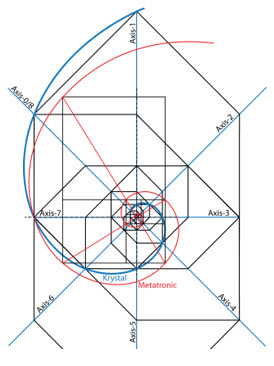To continue reading further sections on this topic please refer to – Spiral Features  or  Spirals & Merkaba.